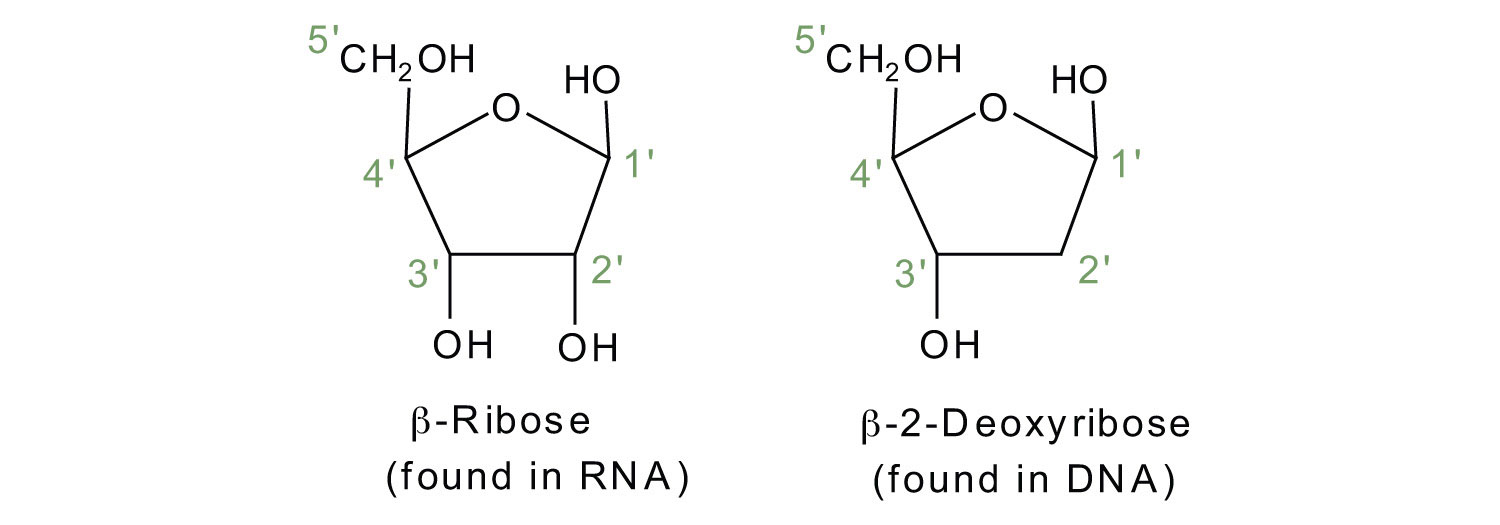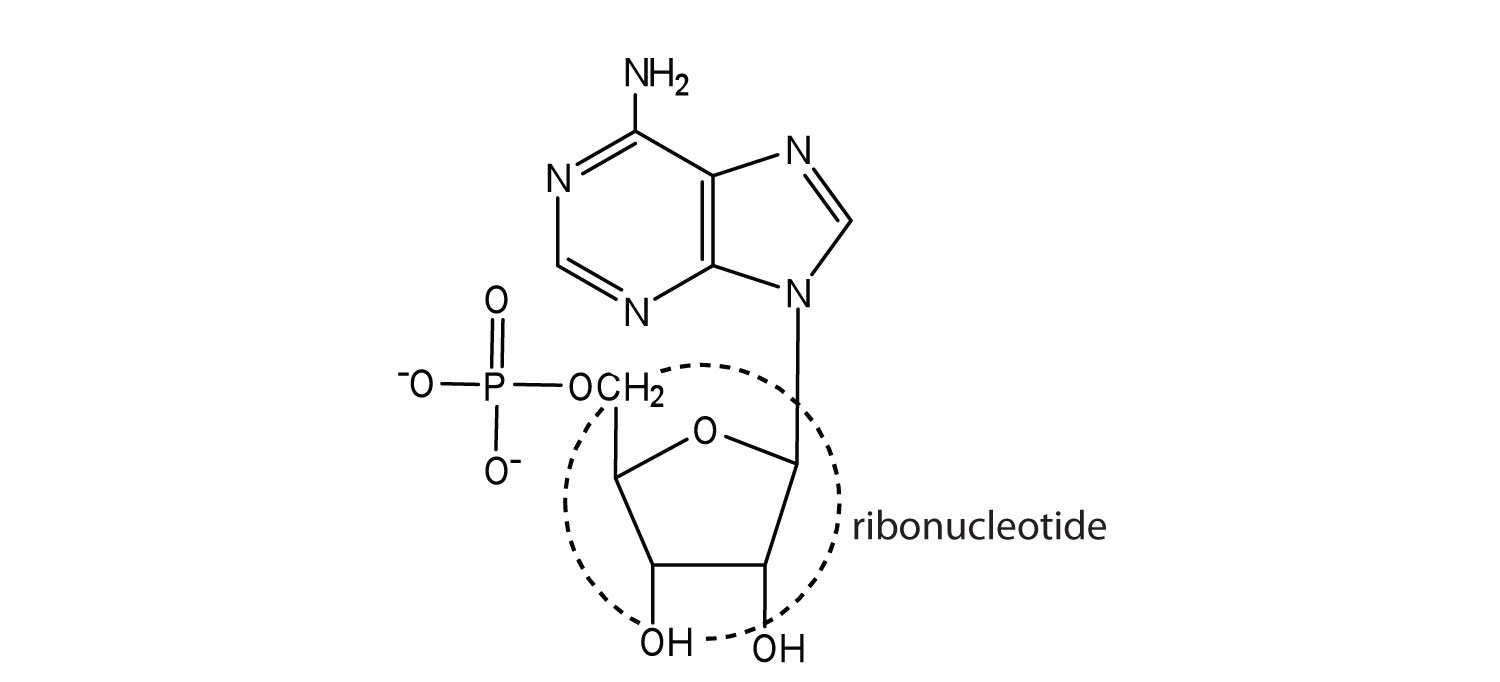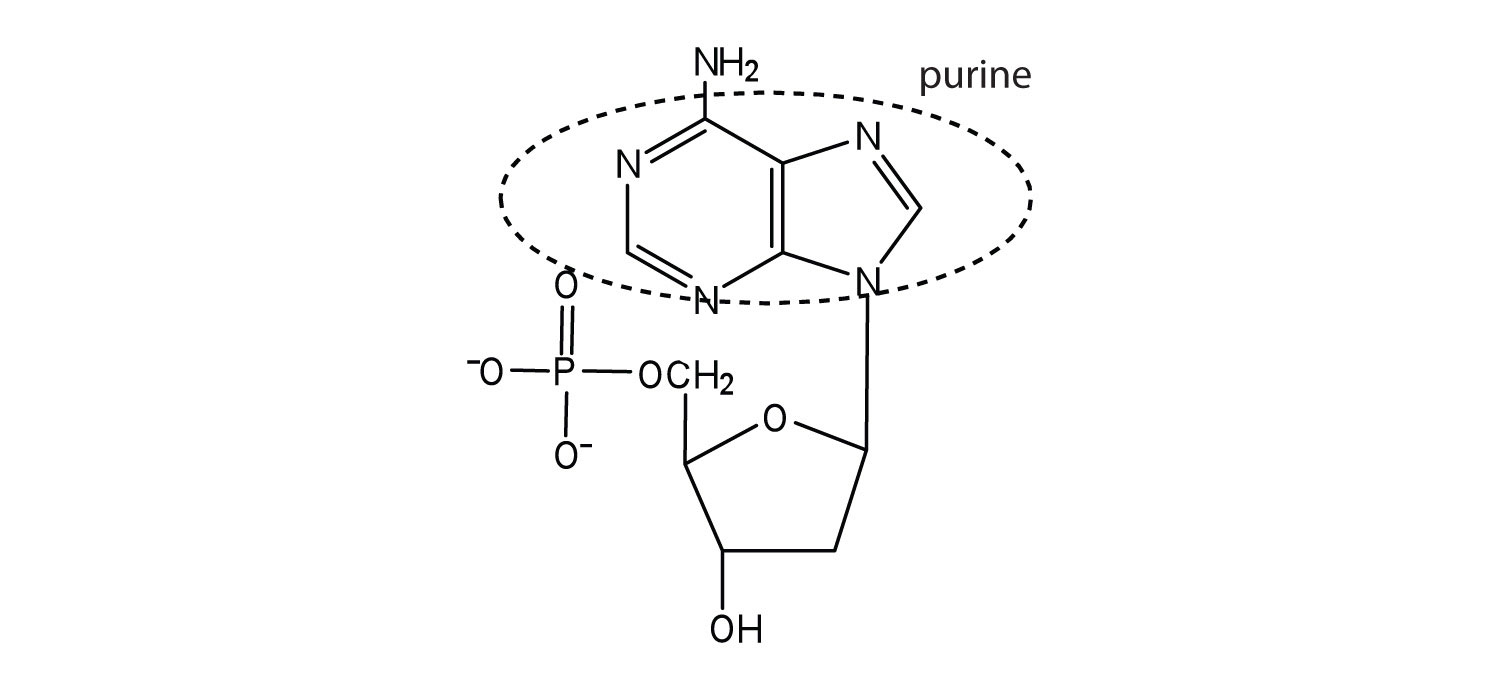# 28.1: Nucleotides and Nucleic Acids

$$\newcommand{\vecs}{\overset { \rightharpoonup} {\mathbf{#1}} }$$ $$\newcommand{\vecd}{\overset{-\!-\!\rightharpoonup}{\vphantom{a}\smash {#1}}}$$$$\newcommand{\id}{\mathrm{id}}$$ $$\newcommand{\Span}{\mathrm{span}}$$ $$\newcommand{\kernel}{\mathrm{null}\,}$$ $$\newcommand{\range}{\mathrm{range}\,}$$ $$\newcommand{\RealPart}{\mathrm{Re}}$$ $$\newcommand{\ImaginaryPart}{\mathrm{Im}}$$ $$\newcommand{\Argument}{\mathrm{Arg}}$$ $$\newcommand{\norm}{\| #1 \|}$$ $$\newcommand{\inner}{\langle #1, #2 \rangle}$$ $$\newcommand{\Span}{\mathrm{span}}$$ $$\newcommand{\id}{\mathrm{id}}$$ $$\newcommand{\Span}{\mathrm{span}}$$ $$\newcommand{\kernel}{\mathrm{null}\,}$$ $$\newcommand{\range}{\mathrm{range}\,}$$ $$\newcommand{\RealPart}{\mathrm{Re}}$$ $$\newcommand{\ImaginaryPart}{\mathrm{Im}}$$ $$\newcommand{\Argument}{\mathrm{Arg}}$$ $$\newcommand{\norm}{\| #1 \|}$$ $$\newcommand{\inner}{\langle #1, #2 \rangle}$$ $$\newcommand{\Span}{\mathrm{span}}$$

##### Objectives

After completing this section, you should be able to

1. outline the relationship between nucleic acids, nucleotides and nucleosides.
2. identify, in general terms, the enzymatic hydrolysis products of nucleosides.
3. explain the structural difference between the sugar components of DNA and RNA.
4. identify by name the four heterocyclic amine bases found in deoxyribonucleotides.
5. identify by name the four heterocyclic amine bases found in ribonucleotides.
6. draw the general structure of a nucleotide and a nucleoside.
7. indicate the nitrogen atom by which a given purine or pyrimidine base attaches to the sugar component in nucleotides and nucleosides.
8. sketch a section of nucleic acid to show how the nucleotide units are joined together.
##### Key Terms

Make certain that you can define, and use in context, the key terms below.

• deoxyribonucleic acid (DNA)
• nucleosides nucleotides
• ribonucleic acid (RNA)
##### Study Notes

The five bases that are found in nucleotides are often represented by their initial letter: adenine, A; guanine, G; cytosine, C; thymine, T; and uracil, U. Note that A, G, C and T occur in DNA; A, G, C and U occur in RNA. You are not required to memorize the structures of these bases, but you must know how each one bonds to the sugar unit in a nucleotide.

To fulfill Objective 6, you should be able to reproduce the figure below.Figure $$\PageIndex{2}$$ The Pyrimidine and Purine Nucleotides below.

The Learning Objective of this Module is to identify the different molecules that combine to form nucleotides.

The repeating, or monomer, units that are linked together to form nucleic acids are known as nucleotides. The deoxyribonucleic acid (DNA) of a typical mammalian cell contains about 3 × 109 nucleotides. Nucleotides can be further broken down to a phosphate group (PO4-3) and a nucleoside which is composed of a aldopentose sugar (a sugar with five carbon atoms), and a heterocyclic purine or pyrimidine base (a base containing nitrogen atoms).

$\mathrm{nucleic\: acids \underset{down\: into}{\xrightarrow{can\: be\: broken}} nucleotides \underset{down\: into}{\xrightarrow{can\: be\: broken}} H_3PO_4 + nitrogen\: base + pentose\: sugar} \tag{28.1.1}$

If the pentose sugar is ribose, the nucleotide is more specifically referred to as a ribonucleotide, and the resulting nucleosides are used in ribonucleic acid (RNA). If the sugar is 2-deoxyribose, the nucleotide is a deoxyribonucleotide, and the nucleoside are used in deoxyribonucleic acid (DNA). The prefix -deoxy implies that there is an oxygen missing from the 2' position of ribose.The nitrogenous bases found in nucleotides are classified as pyrimidines or purines. Pyrimidines are heterocyclic amines with two nitrogen atoms in a six-member ring and include uracil, thymine, and cytosine. Purines are heterocyclic amines consisting of a pyrimidine ring fused to a five-member ring with two nitrogen atoms. Adenine and guanine are the major purines found in nucleic acids (Figure $$\PageIndex{1}$$).  The numbering convention is that primed numbers designate the atoms of the pentose ring, and unprimed numbers designate the atoms of the purine or pyrimidine ring.Figure $$\PageIndex{1}$$: The Nitrogenous Bases Found in DNA and RNA

Nucleosides are formed by a bond between the anomeric C1′ of the pentose sugar and N1 position of the pyrimidine base or the N9 position of the purine base. The addition of a phospate groups at the 5' position of a nucleoside creates a corresponding nucleotide. DNA is made from four deoxyribonucleotides (Cytosine, Thymine, Adenine, Guanine) and RNA is made from four ribonucleotides Cytosine, Uracil, Adenine, Guanine. The names and structures of the major ribonucleotides and deoxyribonucleotides are given in Figure $$\PageIndex{2}$$.Apart from being the monomer units of DNA and RNA, the nucleotides and some of their derivatives have other functions as well. Adenosine diphosphate (ADP) and adenosine triphosphate (ATP), shown in Figure $$\PageIndex{3}$$, have a role in cell metabolism. Moreover, a number of coenzymes, including flavin adenine dinucleotide (FAD), nicotinamide adenine dinucleotide (NAD+), and coenzyme A, contain adenine nucleotides as structural components.Figure $$\PageIndex{3}$$: Structures of Two Important Adenine-Containing Nucleotides

## Primary Structure of Nucleic Acids

Nucleotides are joined together through the phosphate group of one nucleotide connecting in an ester linkage to the OH group on the 3' carbon atom of the sugar unit of a second nucleotide. This unit joins to a third nucleotide, and the process is repeated to produce a long nucleic acid chain (Figure 28.1.4). The backbone of the chain consists of alternating phosphate and sugar units (2-deoxyribose in DNA and ribose in RNA). The purine and pyrimidine bases branch off this backbone.

Like proteins, nucleic acids have a primary structure that is defined as the sequence of their nucleotides. Unlike proteins, which have 20 different kinds of amino acids, there are only 4 different kinds of nucleotides in nucleic acids. For amino acid sequences in proteins, the convention is to write the amino acids in order starting with the N-terminal amino acid. In writing nucleotide sequences for nucleic acids, the convention is to write the nucleotides (usually using the one-letter abbreviations for the bases, shown in Figure 28.1.4) starting with the nucleotide having a free phosphate group, which is known as the 5′ end, and indicate the nucleotides in order. For DNA, a lowercase d is often written in front of the sequence to indicate that the monomers are deoxyribonucleotides. The final nucleotide has a free OH group on the 3′ carbon atom and is called the 3′ end. The sequence of nucleotides in the DNA segment shown in Figure 28.1.4 would be written 5′-dG-dT-dA-dC-3′, which is often further abbreviated to dGTAC or just GTAC.

##### Note

Each phosphate group has one acidic hydrogen atom that is ionized at physiological pH. This is why these compounds are known as nucleic acids.Figure $$\PageIndex{4}$$: Structure of a Segment of DNA. A similar segment of RNA would have OH groups on each C2′, and uracil would replace thymine.

Exercise $$\PageIndex{1}$$

Classify each compound as a pentose sugar, a purine, or a pyrimidine.

2. guanine
3. deoxyribose
4. thymine
5. ribose
6. cytosine

a) purine

b) purine

c) pentose sugar

d) pyrimidine

e) pentose sugar

f) pyrimidine

Exercise $$\PageIndex{2}$$

Identify the three molecules needed to form the nucleotides in each nucleic acid.

1. DNA
2. RNA

a)nitrogenous base (adenine, guanine, cytosine, and thymine), 2-deoxyribose, and H3PO4

b) nitrogenous base (adenine, guanine, cytosine, and uracil), ribose, and H3PO4

Exercise $$\PageIndex{3}$$

For each structure, circle the sugar unit and identify the nucleotide as a ribonucleotide or a deoxyribonucleotide.

1.2.a.b.Exercise $$\PageIndex{4}$$

For each structure, circle the nitrogenous base and identify it as a purine or pyrimidine.

1.2.1.2.